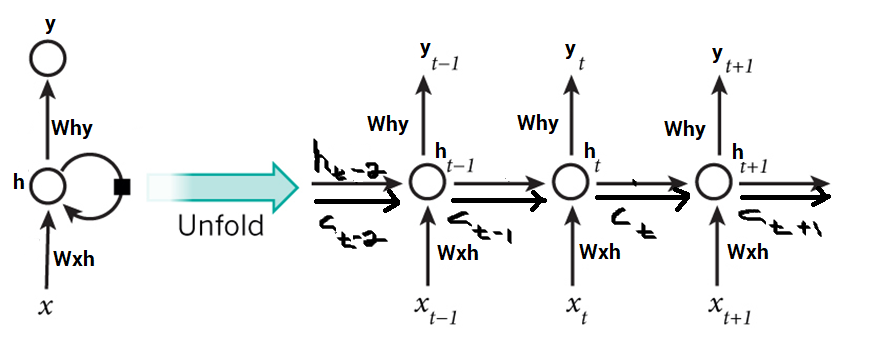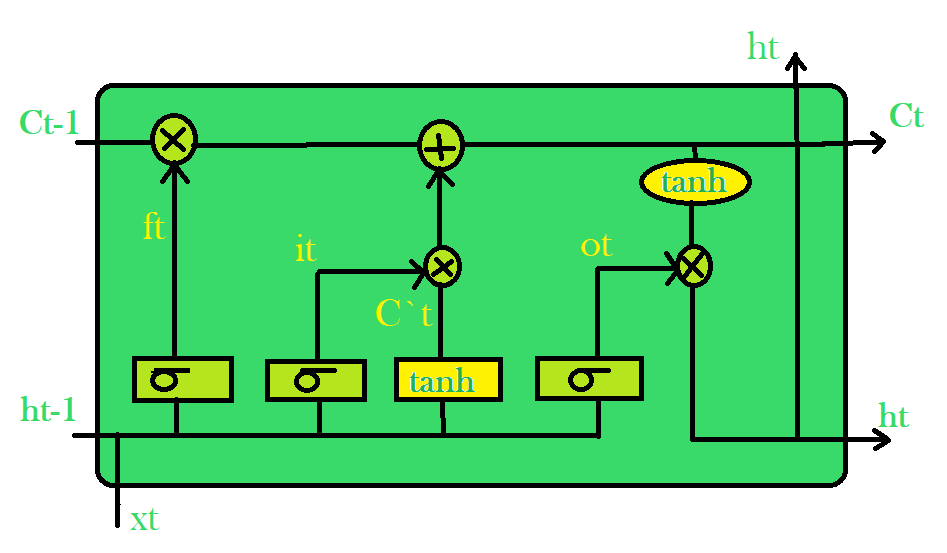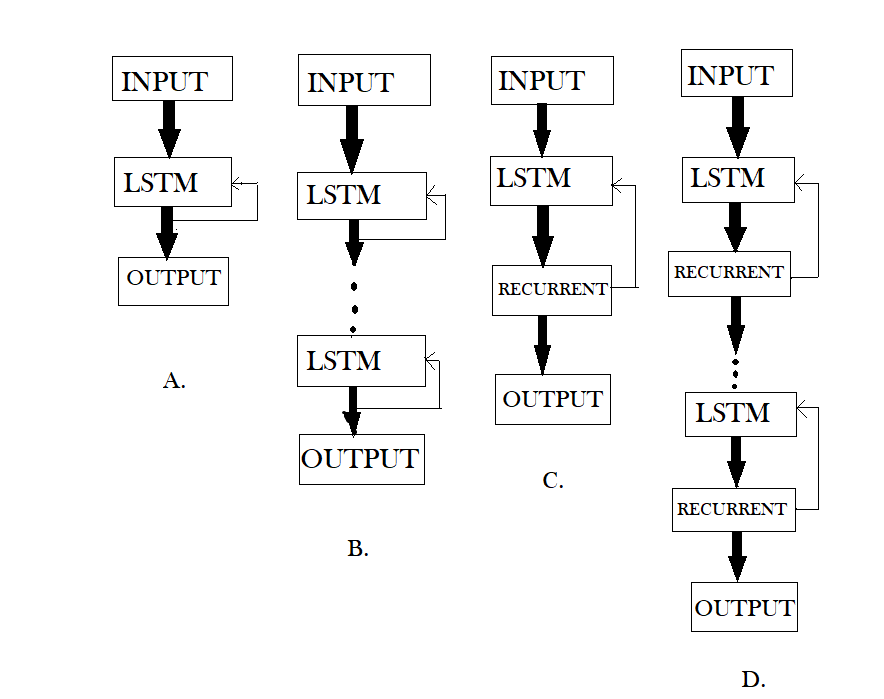# Understanding of LSTM Networks

• Difficulty Level : Expert
• Last Updated : 25 Jun, 2021

This article talks about the problems of conventional RNNs, namely, the vanishing and exploding gradients and provides a convenient solution to these problems in the form of Long Short Term Memory (LSTM). Long Short-Term Memory is an advanced version of recurrent neural network (RNN) architecture that was designed to model chronological sequences and their long-range dependencies more precisely than conventional RNNs. The major highlights include the interior design of a basic LSTM cell, the variations brought into the LSTM architecture, and few applications of LSTMs that are highly in demand. It also makes a comparison between LSTMs and GRUs. The article concludes with a list of disadvantages of the LSTM network and a brief introduction of the upcoming attention-based models that are swiftly replacing LSTMs in the real world.

Introduction:

Attention reader! Don’t stop learning now. Get hold of all the important Machine Learning Concepts with the Machine Learning Foundation Course at a student-friendly price and become industry ready.

LSTM networks are an extension of recurrent neural networks (RNNs) mainly introduced to handle situations where RNNs fail. Talking about RNN, it is a network that works on the present input by taking into consideration the previous output (feedback) and storing in its memory for a short period of time (short-term memory). Out of its various applications, the most popular ones are in the fields of speech processing, non-Markovian control, and music composition. Nevertheless, there are drawbacks to RNNs. First, it fails to store information for a longer period of time. At times, a reference to certain information stored quite a long time ago is required to predict the current output. But RNNs are absolutely incapable of handling such “long-term dependencies”. Second, there is no finer control over which part of the context needs to be carried forward and how much of the past needs to be ‘forgotten’. Other issues with RNNs are exploding and vanishing gradients (explained later) which occur during the training process of a network through backtracking. Thus, Long Short-Term Memory (LSTM) was brought into the picture. It has been so designed that the vanishing gradient problem is almost completely removed, while the training model is left unaltered. Long time lags in certain problems are bridged using LSTMs where they also handle noise, distributed representations, and continuous values. With LSTMs, there is no need to keep a finite number of states from beforehand as required in the hidden Markov model (HMM). LSTMs provide us with a large range of parameters such as learning rates, and input and output biases. Hence, no need for fine adjustments. The complexity to update each weight is reduced to O(1) with LSTMs, similar to that of Back Propagation Through Time (BPTT), which is an advantage.

During the training process of a network, the main goal is to minimize loss (in terms of error or cost) observed in the output when training data is sent through it. We calculate the gradient, that is, loss with respect to a particular set of weights, adjust the weights accordingly and repeat this process until we get an optimal set of weights for which loss is minimum. This is the concept of backtracking. Sometimes, it so happens that the gradient is almost negligible. It must be noted that the gradient of a layer depends on certain components in the successive layers. If some of these components are small (less than 1), the result obtained, which is the gradient, will be even smaller. This is known as the scaling effect. When this gradient is multiplied with the learning rate which is in itself a small value ranging between 0.1-0.001, it results in a smaller value. As a consequence, the alteration in weights is quite small, producing almost the same output as before. Similarly, if the gradients are quite large in value due to the large values of components, the weights get updated to a value beyond the optimal value. This is known as the problem of exploding gradients. To avoid this scaling effect, the neural network unit was re-built in such a way that the scaling factor was fixed to one. The cell was then enriched by several gating units and was called LSTM.

Architecture:

The basic difference between the architectures of RNNs and LSTMs is that the hidden layer of LSTM is a gated unit or gated cell. It consists of four layers that interact with one another in a way to produce the output of that cell along with the cell state. These two things are then passed onto the next hidden layer. Unlike RNNs which have got the only single neural net layer of tanh, LSTMs comprises of three logistic sigmoid gates and one tanh layer. Gates have been introduced in order to limit the information that is passed through the cell. They determine which part of the information will be needed by the next cell and which part is to be discarded. The output is usually in the range of 0-1 where ‘0’ means ‘reject all’ and ‘1’ means ‘include all’.

Hidden layers of LSTM :Each LSTM cell has three inputs,andand two outputsand. For a given time t,is the hidden state,is the cell state or memory,is the current data point or input. The first sigmoid layer has two inputs–andwhereis the hidden state of the previous cell. It is known as the forget gate as its output selects the amount of information of the previous cell to be included. The output is a number in [0,1] which is multiplied (point-wise) with the previous cell stateConventional LSTM:The second sigmoid layer is the input gate that decides what new information is to be added to the cell. It takes two inputsand. The tanh layer creates a vectorof the new candidate values. Together, these two layers determine the information to be stored in the cell state. Their point-wise multiplicationtells us the amount of information to be added to the cell state. The result is then added with the result of the forget gate multiplied with previous cell stateto produce the current cell state. Next, the output of the cell is calculated using a sigmoid and a tanh layer. The sigmoid layer decides which part of the cell state will be present in the output whereas tanh layer shifts the output in the range of [-1,1]. The results of the two layers undergo point-wise multiplication to produce the output ht of the cell.

Variations:

With the increasing popularity of LSTMs, various alterations have been tried on the conventional LSTM architecture to simplify the internal design of cells to make them work in a more efficient way and to reduce the computational complexity. Gers and Schmidhuber introduced peephole connections which allowed gate layers to have knowledge about the cell state at every instant. Some LSTMs also made use of a coupled input and forget gate instead of two separate gates that helped in making both the decisions simultaneously. Another variation was the use of the Gated Recurrent Unit(GRU) which improved the design complexity by reducing the number of gates. It uses a combination of the cell state and hidden state and also an update gate which has forgotten and input gates merged into it.LSTM(Figure-A), DLSTM(Figure-B), LSTMP(Figure-C) and DLSTMP(Figure-D)

1. Figure-A represents what a basic LSTM network looks like. Only one layer of LSTM between an input and output layer has been shown here.
2. Figure-B represents Deep LSTM which includes a number of LSTM layers in between the input and output. The advantage is that the input values fed to the network not only go through several LSTM layers but also propagate through time within one LSTM cell. Hence, parameters are well distributed within multiple layers. This results in a thorough process of inputs in each time step.
3. Figure-C represents LSTM with the Recurrent Projection layer where the recurrent connections are taken from the projection layer to the LSTM layer input. This architecture was designed to reduce the high learning computational complexity (O(N)) for each time step) of the standard LSTM RNN.
4. Figure-D represents Deep LSTM with a Recurrent Projection Layer consisting of multiple LSTM layers where each layer has its own projection layer. The increased depth is quite useful in the case where the memory size is too large. Having increased depth prevents overfitting in models as the inputs to the network need to go through many nonlinear functions.

GRUs Vs LSTMs

In spite of being quite similar to LSTMs, GRUs have never been so popular. But what are GRUs? GRU stands for Gated Recurrent Units. As the name suggests, these recurrent units, proposed by Cho, are also provided with a gated mechanism to effectively and adaptively capture dependencies of different time scales. They have an update gate and a reset gate. The former is responsible for selecting what piece of knowledge is to be carried forward, whereas the latter lies in between two successive recurrent units and decides how much information needs to be forgotten.

Activation at time t:Update gate:Candidate activation:Reset gate:Another striking aspect of GRUs is that they do not store cell state in any way, hence, they are unable to regulate the amount of memory content to which the next unit is exposed. Instead, LSTMs regulate the amount of new information being included in the cell. On the other hand, the GRU controls the information flow from the previous activation when computing the new, candidate activation, but does not independently control the amount of the candidate activation being added (the control is tied via the update gate).

Applications:

LSTM models need to be trained with a training dataset prior to its employment in real-world applications. Some of the most demanding applications are discussed below:

1. Language modelling or text generation, that involves the computation of words when a sequence of words is fed as input. Language models can be operated at the character level, n-gram level, sentence level or even paragraph level.
2. Image processing, that involves performing analysis of a picture and concluding its result into a sentence. For this, it’s required to have a dataset comprising of a good amount of pictures with their corresponding descriptive captions. A model that has already been trained is used to predict features of images present in the dataset. This is photo data. The dataset is then processed in such a way that only the words that are most suggestive are present in it. This is text data. Using these two types of data, we try to fit the model. The work of the model is to generate a descriptive sentence for the picture one word at a time by taking input words that were predicted previously by the model and also the image.
3. Speech and Handwriting Recognition
4. Music generation which is quite similar to that of text generation where LSTMs predict musical notes instead of text by analyzing a combination of given notes fed as input.
5. Language Translation involves mapping a sequence in one language to a sequence in another language. Similar to image processing, a dataset, containing phrases and their translations, is first cleaned and only a part of it is used to train the model. An encoder-decoder LSTM model is used which first converts input sequence to its vector representation (encoding) and then outputs it to its translated version.

Drawbacks:

As it is said, everything in this world comes with its own advantages and disadvantages, LSTMs too, have a few drawbacks which are discussed as below:

1. LSTMs became popular because they could solve the problem of vanishing gradients. But it turns out, they fail to remove it completely. The problem lies in the fact that the data still has to move from cell to cell for its evaluation. Moreover, the cell has become quite complex now with the additional features (such as forget gates) being brought into the picture.
2. They require a lot of resources and time to get trained and become ready for real-world applications. In technical terms, they need high memory-bandwidth because of linear layers present in each cell which the system usually fails to provide for. Thus, hardware-wise, LSTMs become quite inefficient.
3. With the rise of data mining, developers are looking for a model that can remember past information for a longer time than LSTMs. The source of inspiration for such kind of model is the human habit of dividing a given piece of information into small parts for easy remembrance.
4. LSTMs get affected by different random weight initialization and hence behave quite similar to that of a feed-forward neural net. They prefer small weight initialization instead.
5. LSTMs are prone to overfitting and it is difficult to apply the dropout algorithm to curb this issue. Dropout is a regularization method where input and recurrent connections to LSTM units are probabilistically excluded from activation and weight updates while training a network.

My Personal Notes arrow_drop_up
Recommended Articles
Page :